# How to visualize the results of a conStruct analysis

## Visualize results

This document describes the use of the functions included in the conStruct package for visualizing analysis outputs. For more information on how to run a conStruct analysis, see the companion vignette for running conStruct.

Throughout, this vignette will make use of the example data output objects generated by a conStruct run:

library(conStruct)
data(data.block)

## Make all the plots

If the make.figs is set to TRUE in a conStruct run, the run will finish by calling the function make.all.the.plots. As the name implies, this function makes all the relevant plots from a set of conStruct results:

• STRUCTURE plot
• Admixture pie plot
• Model fit plot
• Layer covariance functions plot
• Trace plots for relevant MCMC quantities including:
• the log posterior density
• nugget parameters
• gamma parameter
• layer-specific parameters
• admixture proportions

More information is available in the documentation for the function, which you can view using the command:

help(make.all.the.plots)

If you deleted the output plots from an analysis, or if you set make.figs to FALSE to avoid making them in the first place, you can make them by calling the make.all.the.plots function. The arguments you have to specify are a conStruct.results output object and a data.block output object, both of which are automatically generated and saved when you execute a conStruct analysis. You must also specify a prefix, which will be prepended to all output pdf file names. If you choose, you can specify a the colors you want each layer to be plotted in; if none are specified, the function will use its own internal vector of colors, which I think look nice but are otherwise arbitrary.

An example call to make.all.the.plots using the example output data objects loaded above is shown below.

make.all.the.plots(conStruct.results = conStruct.results,
data.block = data.block,
prefix = "example",
layer.colors = NULL)
# generates a bunch of pdf figures

## Visualizing estimated admixture proportions

Generally, users are most interested in the estimated admixture proportions for each sample. These are commonly visualized using STRUCTURE plots and pie plots. Functions for both are included in the package, and their use is detailed below.

### STRUCTURE plots

Probably the most common method for visualizing admixture proportions is using a stacked bar plot (commonly called a STRUCTURE plot after the model-based clustering method STRUCTURE).

Users can generate a STRUCTURE plot for their data using the command make.structure.plot, (see documentation at help(make.structure.plot)). This function takes as its principal argument the estimated admixture proportions and makes a STRUCTURE plot in the plotting window. An example is given below.

First, we load the conStruct.results data output object and, for convenience, assign the maximum a posteriori admixture parameter estimates to a variable with a shorter name:

load("my_conStruct.results.Robj")

# assign the MAP admixture proportions from
#   the first MCMC chain to a variable
#   with a new name

admix.props <- conStruct.results$chain_1$MAP$admix.proportions Now we can visualize the results: # make a STRUCTURE plot using the # maximum a posteriori (MAP) estimates # from the first chain of a conStruct run make.structure.plot(admix.proportions = admix.props)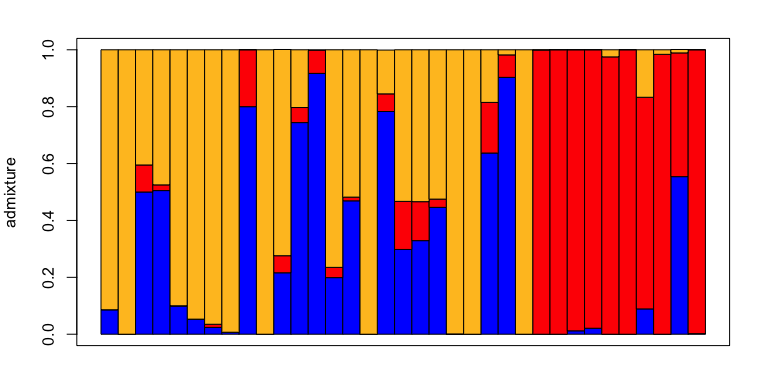#### Order STRUCTURE plots The function also includes a variety of options for tweaking the order of the plotted samples.  # order by membership in layer 1 make.structure.plot(admix.proportions = admix.props, sort.by = 1)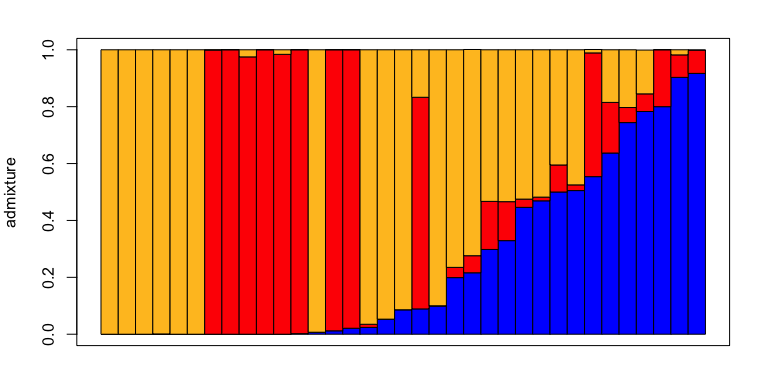# re-order the stacking order of the layers make.structure.plot(admix.proportions = admix.props, layer.order = c(2,1,3), sort.by = 2)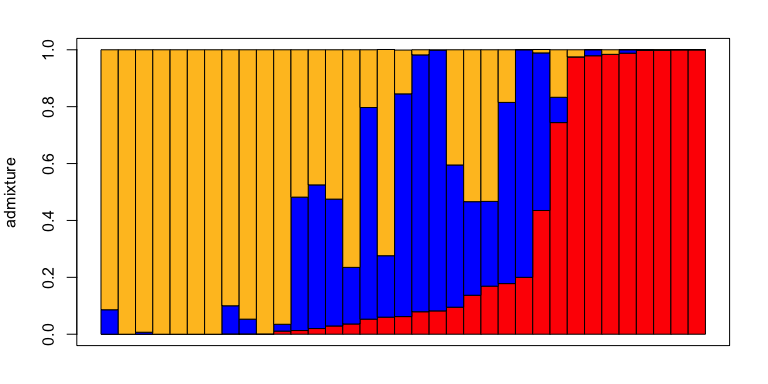# provide a custom sample ordering # in this case by sample latitude make.structure.plot(admix.proportions = admix.props, sample.order = order(data.block$coords[,2]))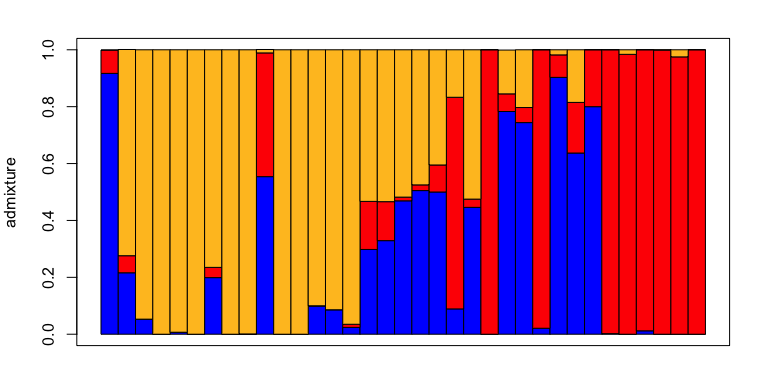# add sample names
make.structure.plot(admix.proportions = admix.props,
sample.names = row.names(data.block$coords), mar = c(4.5,4,2,2))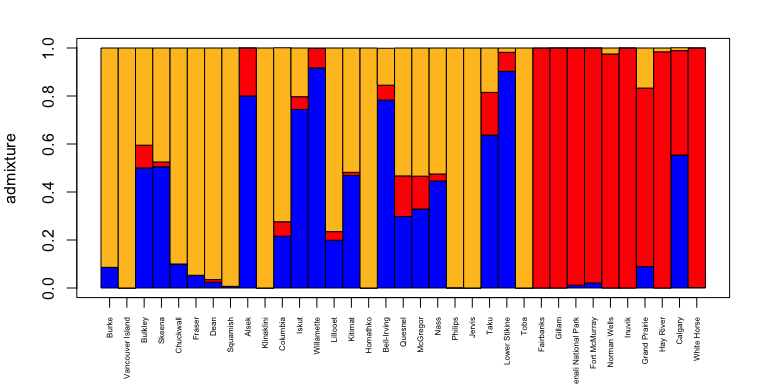### ADMIXTURE pie plots It is often also useful to visualize estimated admixture proportions in a spatial context by plotting them on a map. The most common way to do this is to plot a pie plot at the sampling location of each sample, in which each modeled layer gets its own slice of the pie (K wedges), and the size of each slice in the pie is proportional to the sample’s admixture proportion in that layer. Users can make an admixture pie plot with their own data using the command make.admix.pie.plot (see documentation at help(make.admix.pie.plot). This function takes as its principal arguments the estimated admixture proportions and the sample coordinates, then makes an admixture pie plot in the plotting window. An example is given below: # make an admix pie plot using the # maximum a posteriori (MAP) estimates # from the first chain of a conStruct run make.admix.pie.plot(admix.proportions = admix.props, coords = data.block$coords)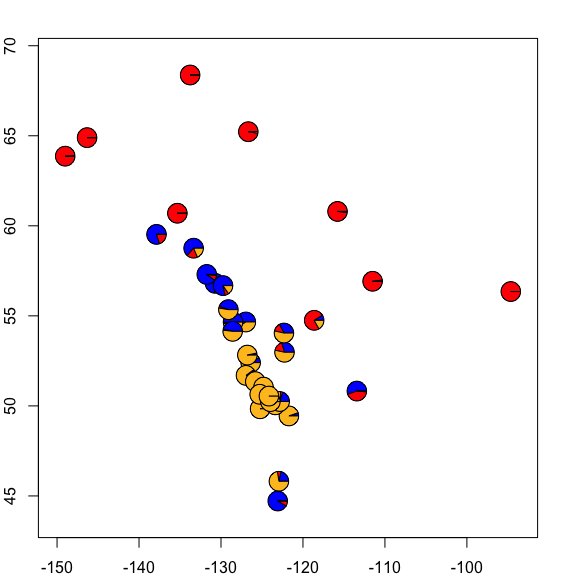# increase pie chart size
make.admix.pie.plot(admix.proportions = admix.props,
coords = data.block$coords, radii = 4)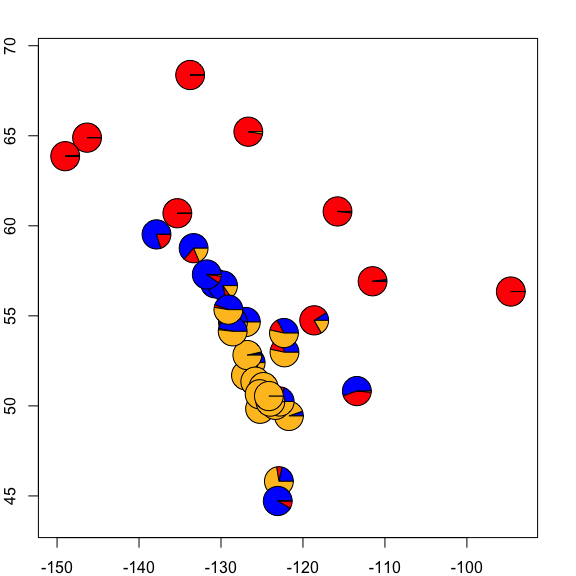# zoom in on a subsection of the map make.admix.pie.plot(admix.proportions = admix.props, coords = data.block$coords,
x.lim = c(-130,-120),
y.lim = c(49,56))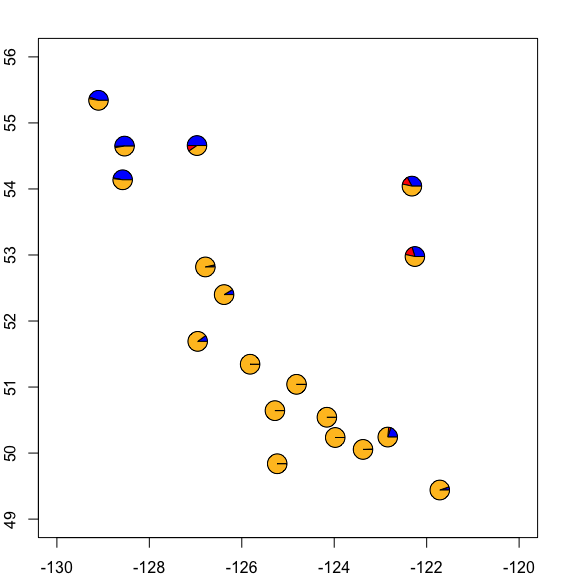#### Pie plot on a map

Users can also add the pie plot directly to a map of their own creation using the make.admix.pie.plot by setting the add argument to TRUE. E.g.,


# add pie plot to an existing map

# make the desired map
maps::map(xlim = range(data.block$coords[,1]) + c(-5,5), ylim = range(data.block$coords[,2])+c(-2,2), col="gray")

# add the admixture pie plot
make.admix.pie.plot(admix.proportions = admix.props,
coords = data.block\$coords,
add = TRUE)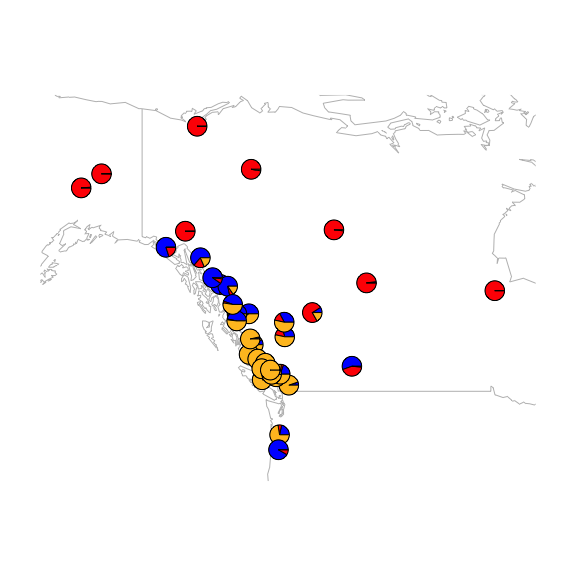## Comparing two conStruct runs

If you’ve run multiple conStruct analyses you may want to visually compare them. Although you could always just open up both sets of output pdfs, label-switching between independent runs can make visual comparisons difficult. Label-switching different models have the same, or very similar, estimated admixture proportions, but with a different permutation of layer labels (e.g., Layer 1 in run 1, and Layer 3 in run 2). To enable easy comparison between a pair of conStruct runs, you can use the function compare.two.runs.

To do so, you need to specify to sets of conStruct.results output R objects, as well as the data.block objects associated with each run. Independent runs with the same model can be compared, as can analyses run with different models (e.g., spatial vs. nonspatial) or different values of K. The only restriction is that if the user is comparing two models run with different values of K, the run with the smaller value should be specified first (conStruct.results2). Documentation for compare.two.runs can be found using the command help(compare.two.runs). Example usage is shown below:

# load output files from a run with
#   the spatial model and K=4
load("spK4.conStruct.results.Robj")
load("spK4.data.block.Robj")

# assign to new variable names
spK4_cr <- conStruct.results
spK4_db <- data.block

# load output files from a run with
#   the spatial model and K=3
load("spK3.conStruct.results.Robj")
load("spK3.data.block.Robj")

# assign to new variable names
spK3_cr <- conStruct.results
spK3_db <- data.block

# compare the two runs
compare.two.runs(conStruct.results1=spK3_cr,
data.block1=spK3_db,
conStruct.results2=spK4_cr,
data.block2=spK4_db,
prefix="spK3_vs_spK4")

# generates a bunch of pdf figures